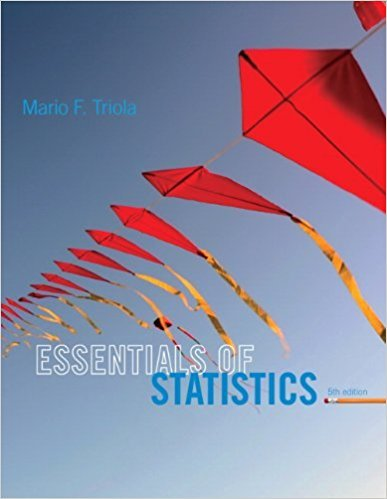×
×

# Solutions for Chapter 11.3: Contingency Tables## Full solutions for Essentials of Statistics | 5th Edition

ISBN: 9780321924599Solutions for Chapter 11.3: Contingency Tables

Solutions for Chapter 11.3
4 5 0 428 Reviews
26
2
##### ISBN: 9780321924599

Since 22 problems in chapter 11.3: Contingency Tables have been answered, more than 14883 students have viewed full step-by-step solutions from this chapter. Chapter 11.3: Contingency Tables includes 22 full step-by-step solutions. Essentials of Statistics was written by and is associated to the ISBN: 9780321924599. This textbook survival guide was created for the textbook: Essentials of Statistics, edition: 5. This expansive textbook survival guide covers the following chapters and their solutions.

Key Statistics Terms and definitions covered in this textbook
• 2 k factorial experiment.

A full factorial experiment with k factors and all factors tested at only two levels (settings) each.

• 2 k p - factorial experiment

A fractional factorial experiment with k factors tested in a 2 ? p fraction with all factors tested at only two levels (settings) each

• Bimodal distribution.

A distribution with two modes

• Block

In experimental design, a group of experimental units or material that is relatively homogeneous. The purpose of dividing experimental units into blocks is to produce an experimental design wherein variability within blocks is smaller than variability between blocks. This allows the factors of interest to be compared in an environment that has less variability than in an unblocked experiment.

• Cause-and-effect diagram

A chart used to organize the various potential causes of a problem. Also called a ishbone diagram.

• Central tendency

The tendency of data to cluster around some value. Central tendency is usually expressed by a measure of location such as the mean, median, or mode.

• Combination.

A subset selected without replacement from a set used to determine the number of outcomes in events and sample spaces.

• Conditional probability distribution

The distribution of a random variable given that the random experiment produces an outcome in an event. The given event might specify values for one or more other random variables

• Control limits

See Control chart.

• Degrees of freedom.

The number of independent comparisons that can be made among the elements of a sample. The term is analogous to the number of degrees of freedom for an object in a dynamic system, which is the number of independent coordinates required to determine the motion of the object.

• Deming’s 14 points.

A management philosophy promoted by W. Edwards Deming that emphasizes the importance of change and quality

• Eficiency

A concept in parameter estimation that uses the variances of different estimators; essentially, an estimator is more eficient than another estimator if it has smaller variance. When estimators are biased, the concept requires modiication.

• Estimate (or point estimate)

The numerical value of a point estimator.

• Factorial experiment

A type of experimental design in which every level of one factor is tested in combination with every level of another factor. In general, in a factorial experiment, all possible combinations of factor levels are tested.

• Finite population correction factor

A term in the formula for the variance of a hypergeometric random variable.

• Fisher’s least signiicant difference (LSD) method

A series of pair-wise hypothesis tests of treatment means in an experiment to determine which means differ.

• Fraction defective

In statistical quality control, that portion of a number of units or the output of a process that is defective.

• Frequency distribution

An arrangement of the frequencies of observations in a sample or population according to the values that the observations take on

• Gamma function

A function used in the probability density function of a gamma random variable that can be considered to extend factorials

• Generating function

A function that is used to determine properties of the probability distribution of a random variable. See Moment-generating function

×